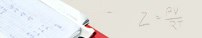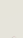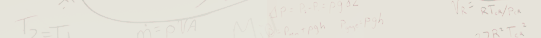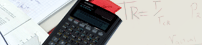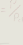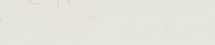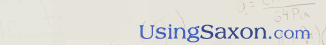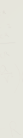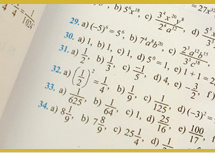ALGEBRA 2, 2 nd or 3 rd Edition DVD SERIES (129  Lessons plus 2 Review Lessons)Introduction: Prerequisites for the Course - How To Use The Math Book - Expectations of Students. Review A: Review of - The Language of Geometry - Use of the Absolute Value - Algebraic Definitions. Review B: Review of Area and Volume. Lesson 1: Introduction of Transversals of Parallel Lines & Proportional Segments, and Review of Polygons &Triangles, Lesson 2: Working with Positive & Negative Exponents - Using the Product & Power Rules with Exponents - Circles Lesson 3: Adding Like Terms - Evaluating Algebraic Expressions. Lesson 4: Using the Distributive Property - Solving Equations having only One Unknown. Lesson 5: Solving Word Problems Using Equations - Finding Fractional Parts of Quantities. Lesson 6: Solving Equations Containing Decimals - and Solving Word Problems involving Consecutive Integers Lesson 7: Solving Word Problems involving Percents - Geometric Equations containing Algebraic Terms. Lesson 8: Review of Polynomials - Graphing Linear Equations Using the "Slope-Intercept Method." Lesson 9: More Challenging Percent Word Problems. Lesson 10: Application of the Pythagorean Theorem. Lesson 11: Adding Algebraic Fractions - Defining a Central Angle & Inscribed Angles. Lesson 12: Using Graphs to Determine the Equation of Straight Lines. Lesson 13: Using Substitution to Solve Equations with Two Unknown Variables (Part I) - Finding the Area of an Isosceles Triangle. Lesson 14: Finding the Equation of a Line Using Two Points or With a Given Slope. Lesson 15: Using Elimination to Solve Equations with Two Unknown Variables. Lesson 16: Multiplying Polynomials - Dividing Polynomials (Part I). Lesson 17: Working with Subscripted Variables - The Algebraic Relationship of Angles Lesson 18: Working with Ratio & Proportion Word Problems - Solving Similar Triangles (Part I). Lesson 19: Word Problems Involving Money or Value - Geometric Relationships. Lesson 20: Simplifying Radicals - Equations of Parallel Lines. Lesson 21: Scientific Notation - Using Statements of Equality with Ratios and Proportions. Lesson 22: Solving Uniform Motion Word Problems involving Equal Distances (Part I) - Solving Similar Triangles (Part II). Lesson 23: Solving Two Equations with Two Unknowns by Graphing the Lines. Lesson 24: Working With Equations Containing Fractions - Overlapping Triangles Part I (Another Form of Similar Triangles). Lesson 25: Introduction to Algebraic Factoring - Relationships of Angles and Sides Formed by Parallel Lines Lesson 26: Introduction to Trinomial Factoring - Overlapping Triangles Part II Lesson 27: Using a Common Denominator in Trinomial Factoring. Lesson 28: Simplifying Abstract, Complex Fractions (Part I) - Working With Radicals in the Denominator. Lesson 29: Uniform Motion Problems involving Total Distance (Part II) Lesson 30: Euclid's "Deductive Reasoning" - Working with Vertical Angles - Angles Formed by Parallel Lines & Transversals. Lesson 31: Application of Negative Reciprocals - Slopes of Perpendicular Lines - Defining Remoter Interior Angles Lesson 32: Application of the "Quotient Theorem" to Radicals - Applying Congruency to Triangles - Transformations. Lesson 33: Simplifying More Advanced Abstract, Complex Fractions (Part II). Lesson 34: Solving Uniform Motion Problems involving Unequal Distances (Part III). Lesson 35: Defining Angles in Polygons - What Are Inscribed Quadrilaterals? - Introduction to Fractional Exponents (Part I). Lesson 36: Multiplication and Division of Rational Expressions (Factoring and Simplifying). Lesson 37: Word Problems Involving the Ratios of Chemical Compounds - Relationship of Angles & Sides in Parallelograms. Lesson 38: Using the Power Rule with Exponents in Algebraic Terms - Factoring and Finding the Roots of Trinomials - Zero? Lesson 39: The Difference of Two Squares - Proof of the Parallelogram - The Relationship of Sides and Angles of a Rhombus Lesson 40: More Abstract, Fractional Equations (Part III). Lesson 41: Working with U.S. standard Units of Measurement and Converting Them using Unit Multipliers. Lesson 42: Using Scientific Notation to Estimate Very Large and Very Small Numbers. Lesson 43: Introduction to Basic Trigonometry. (Using the Sine, Cosine, and Tangent) Lesson 44: Working with and Solving Right Triangles. Lesson 45: Review of Factoring the "Difference of Two Squares." Lesson 46: Working with radical Expressions (Part II) - "Nested" Radicals and Fractional Exponents (Part II). Lesson 47: Unit Conversions Involving Rates - "Nested" Radicals and Fractional Exponents (Part III) Lesson 48: Solving More Advanced Equations Involving Radical Expressions. Lesson 49: Finding the "Y-Intercept" in Linear Equations - Transversals and Trigonometry. Lesson 50: Solving Quadratic Equations by "Completing the Square." Lesson 51: Working with the Set of Complex Numbers - Imaginary Numbers - Euler's Notation. Lesson 52: Word Problems Involving Chemical Mixtures (Part I). Lesson 53: Using Unit Multipliers to Convert Metric Units to US and US to Metric - Chemical Compounds (Part II). Lesson 54: Vectors - Converting Polar Coordinates to Rectangular Coordinates - Solving Similar Triangles (Part (III) Lesson 55: More Advanced Abstract Equations - Solving Word Problems Involving Quadratic Equations. Lesson 56: The Relationship of Sides and Angles to Circles. Lesson 57: Word Problems Involving the "Combined" or "Ideal" Gas Laws (Part I). Lesson 58: Working with "Lead" Coefficients" in Completing the Square. Lesson 59: Graphing Experimental Data (Part I) - Equations with Decimals & Fractions - Converting Rectangular to Polar Form. Lesson 60: Working with both Direct and Inverse Ratios and Proportions. Lesson 61: Word Problems Involving Chemical Mixtures (Part II). Lesson 62: Finding the Complex Roots of Quadratic Equations (a +/- bi). Lesson 63: Adding Vectors. Lesson 64: More Advanced Abstract Complex Fractions (Part III) Lesson 65: Solving Equations with Subscripted Variables using Substitution (Part II). Lesson 66: Changing the Signs of Fractions - Working with 30-60-90 degree Triangles. Lesson 67: Solving fractions that have Multiple Radical Denominators Lesson 68: Scientific Notation - Expressing Powers and Roots on a Scientific Calculator (Casio fx360 solar - \$9.99). Lesson 69: Word Problems Involving the "Combined" or "Ideal" Gas Laws (Part II). Lesson 70: More Advanced Abstract Complex Equations (Part IV). Lesson 71: Factoring Trinomials using the Quadratic Formula. Lesson 72: Graphing Experimental Data (Part II) - Defining Negative Angles. Lesson 73: Multiple Radical Denominators (Part II) Lesson 74: More Word Problems Involving Distance (Part IV) Lesson 75: Factoring Trinomials while Applying the Rules for changing the "Signs of Fractions." Lesson 76: Solving three Equations with Three Unknown Variables - Defining a Negative Vector. Lesson 77: More Advanced Radical Equations (Part III) - Solving Equations with Multiple Radicals. Lesson 78: Using Trigonometry to calculate resulting "Force Vectors." Lesson 79: Calculating Metric Volume - Working with 45-45-90 degree Triangles. Lesson 80: Working with Direct and Inverse Variation in Ratios and Proportion (Part II) Lesson 81: Multiplying and Dividing with Complex Numbers. Lesson 82: Simplifying Abstract, Complex fractions (Part IV). Lesson 83: Rules for Working with Exponents that Are Variables. Lesson 84: Defining the Three Possible Solutions for Equations Lesson 85: Solving for the variables in Systems of "Non-Linear" Equations (Part I). Lesson 86: Defining "Trichotomy" & "Transitivity" - Irrational Roots (Part I) Lesson 87: Definition and Application of the "Slope" formula in Linear Equations. Lesson 88: Application of the "Distance" Formula - Problems Dealing with the Ideal Gas Law (Part III) Lesson 89: Mathematical "Conjunctions" and "Disjunctions" - The Relationship of Products of "Chords" and "Secants" in Circles. Lesson 90: Solving systems of Three Equations containing Three Unknown Variables (Part I). Lesson 91: Solving systems of Linear "Inequalities" Lesson 92: Solving Word Problems involving Going both against & with River Currents (Part I) Lesson 93: Using the "Discriminant" from the Quadratic Equation. Lesson 94: Defining "Functions", Functional Notation", and "Dependant" and "Independent" Variables - Lesson 95: Solving More Advanced Systems of Non-Linear Equations (Part II) Lesson 96: Equations using Joint, Inverse, Direct and Indirect variations of relationship (Part II) -Irrational Roots (Part II) Lesson 97: Using Substitution in Solving Systems of Two Equations with Two Unknowns (Part III). Lesson 98: Finding Fractional Distances between Numbers. Lesson 99: Applying "Conjunctions and "Disjunctions" to Absolute Values - Absolute Values less than Zero - Definition of "Null." Lesson 100: Graphing non-linear functions called "Parabolas." Lesson 101: Defining "Selling Price, Purchase Price" and "Mark-Up." Lesson 102: Working with "Functional Notation" - Finding the "Sums" and "Products" of Functions. Lesson 103: Dividing Polynomials (Part II) Lesson 104: Reviewing the set of Complex Numbers - The subset of Real Numbers - Working with Repeating Decimal Digits. Lesson 105: Advanced Factoring of Trinomials. Lesson 106: More on Solving Systems of Three Equations with Three Unknowns (Part II) Lesson 107: Word Problems Involving the Place Values of Numbers. Lesson 108: Factoring the Sum and Difference of Two Cubes. Lesson 109: More on Working with Exponents Containing Fractions (Part IV). Lesson 110: Graphing Quadratic Inequalities (Part I) Lesson 111: Word Problems Containing Statements of Equality. Lesson 112: Graphing Quadratic Inequalities (Part II) Lesson 113: Logarithms (Part I) Lesson 114: Graphing Non-Linear Inequalities. Lesson 115: Solving Exponential Functions - Working with Compound Interest. Lesson 116: Probability and Permutations. Lesson 117: Using Symbols for Sets of Numbers in Describing Functions. Lesson 118: Logarithms (Part II) Lesson 119: Inequalities Involving Absolute Values. Lesson 120: Word Problems Comparing Time Differences. Lesson 121: Graphing Rational Inequalities. Lesson 122: Logarithms (Part III) - Symbols for the Union & Intersection of Number Sets - Venn Diagrams Lesson 123: Location of Points from both a Given Line and a Given Point - Basic Construction using Straight Edge and Compass. Lesson 124: Proofs of Triangles using SSS, AAS, SAS, and HL - (Part I) Lesson 125: Two Column "Formal Proofs" (Part I) - Relationships between Chords, Tangents, and Circles. Lesson 126: Revisiting Quadrilaterals - Relationship between Chords, Angles and Circles - Two Column Proofs (Part II). Lesson 127: Visualizing "Solid" Geometry - Two Column Proofs (Part III) Lesson 128: Circumscribed, Inscribed, and Central Angles and Triangles Lesson 129: Revisiting Stem & Leaf Plots - Standard Deviation. Home | Review the book | Purchase book | Testimonials | Newsletter | Contact Us © 2006 - 2022 AJ Publishers, LLC